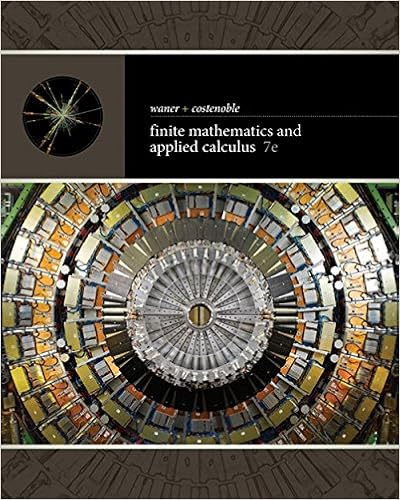# Chapter 3 summary key.doc - Chapter 3 3.1 Sampling...

• 22
• 88% (52) 46 out of 52 people found this document helpful

This preview shows page 1 - 4 out of 22 pages.

##### We have textbook solutions for you!
The document you are viewing contains questions related to this textbook.The document you are viewing contains questions related to this textbook.
Chapter 9 / Exercise 70
Finite Mathematics and Applied Calculus
Costenoble/WanerExpert Verified
Chapter 33.1 Sampling DitributionsExample:For each of the following, state whether the quantity described is a parameter or a statistic, and give the correct notation.Average household income for all houses in the US, using data from the US census Parameter, (symbol µ)Correlation between height and weight for players on the 2014 Brazil world cup team, using data from all 23 players on the rosterParameter (symbol ρ) Proportion of people who use an electric toothbrush, using data from a sample of300 adults Statistic (symbol Example 1: Using Search Engines on the InternetA 2012 survey of a random sample of 2253 US adults found that 1,329 of them reported using a search engine (such as Google) every day to find information on the Internet. a). Find the relevant proportion and give the correct notation with it. b). Is your answer to part (a) a parameter or a statistic? )Statistic
##### We have textbook solutions for you!
The document you are viewing contains questions related to this textbook.The document you are viewing contains questions related to this textbook.
Chapter 9 / Exercise 70
Finite Mathematics and Applied Calculus
Costenoble/WanerExpert Verified
c). Give notation for and define the population parameter that we estimate using the Result of part (a).
Example 2: Number of Books Read in a YearA survey of 2,986 Americans ages 16 and older found that 80% of them read at least one book in the last year. Of these book readers, the mean number of books read in the last year is 17 while the median number of books read in the last year is 8. a). How many “book readers” (defined as reading at least one book in the past year) were included in the sample?
b). Why might the mean and median be so different? Using the information given about the mean and median number of books read in a year, what is the likely shape of the distribution of number of books read in a year by book readers?
c). Give the correct notation for the value “17” in the information above. Is this value a parameter or a statistic?
.d). Give notation for and define the population parameter that we estimate using the result of part (c).
[Emphasize that we are estimating the mean of the population.]
•••